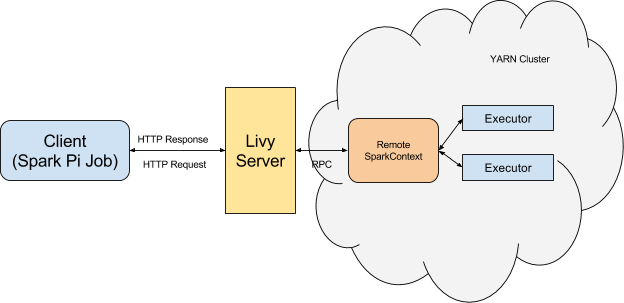# Using the Livy API to run Spark jobs

Using the Livy API to run Spark jobs is similar to using the original Spark API.

The following two examples calculate Pi.

Calculate Pi using the Spark API:

```def sample(p):
x, y = random(), random()
return 1 if x*x + y*y < 1 else 0
count = sc.parallelize(xrange(0, NUM_SAMPLES)).map(sample) \
.reduce(lambda a, b: a + b)```

Calculate Pi using the Livy API:

```def f(_):
x = random() * 2 - 1
y = random() * 2 - 1
return 1 if x ** 2 + y ** 2 <= 1 else 0
def pi_job(context):
count = context.sc.parallelize(range(1, samples + 1), slices).map(f).reduce(add)
return 4.0 * count / samples```

There are two main differences between the two APIs:

• When using the Spark API, the entry point (SparkContext) is created by user who wrote the code. When using the Livy API, SparkContext is offered by the framework; the user does not need to create it.

• The client submits code to the Livy server through the REST API. The Livy server sends the code to a specific Spark cluster for execution.

Architecturally, the client creates a remote Spark cluster, initializes it, and submits jobs through REST APIs. The Livy server unwraps and rewraps the job, and then sends it to the remote SparkContext through RPC. While the job runs the client waits for the result, using the same path. The following diagram illustrates the process: